# Generation of random sequences and Time sequences

1 müşteri puanına dayanarak 5 üzerinden 5.00 puan aldı
(1 müşteri yorumu)

\$ 200,00

Stokta yok

Kategoriler: Etiketler:

## Açıklama

Generation of random sequences: While deterministic signals such as square pulses, sinc waveforms, sinuses and cosines are used in specific applications, almost all other real-life signals from econometric series to radar returns, from genetic codes to multimedia signals in consumer electronics are information-bearing random signals.

• Time sequences: Generate a sequence of 1000 equally spaced samples of a Gauss-Markov process using the recursive relation: . Here assume that , and  is a sequence of independent identically distributed Gaussian random variables. You can use the randn function in MATLAB to generate zero-mean, unit-variance random variables.  Below is given a low-pass filter (a>0) excited by a white noise sequence, and the filter has a real pole at z= a.  Plot the output waveform for the following values of a: a= 0.5, a = 0.95, a = 0.995.  Comment on the effect of the selection of a on the resulting time sequence.
• Autocorrelation function: In order to estimate the autocorrelation function of this discrete process, one can apply the formula: . However, since we have a finite number of samples an approximation would be . Notice we shorten the data and consider only the overlapping samples in the window starting at n=1 and the window starting at n=m+1.   The theoretical autocorrelation for such a signal model is also known as: , where is the input process variance.  Plot the autocorrelation sequence in three separate graphs for the values of a= 0.5, a = 0.95, a = 0.995, but superpose in each graph the analytical and estimated  and  correlation functions.
• Power Spectrum: Using the Wiener-Khinchine theorem, we can find the power spectrum in two different ways. Then do the following:
1. Estimate , where , for a=0.95 and N=512
2. Estimate again as above, but this time averaging ten estimates, each obtained from a different time sequence. In other words, you must use the recursion , ten times, and average the results, i.e., . The estimates are noisy and random, and to improve and smooth the estimates, we must calculate the quantities several times (let us say 10 times) and average them.
• We also know the analytical value of , where is the power spectral density of the input white noise, hence, , and

Plot the power spectra in these three cases and comment on each of them.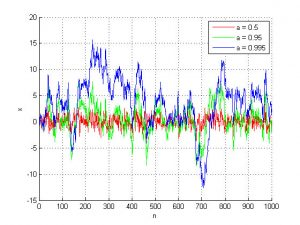MATLAB Handle Graphics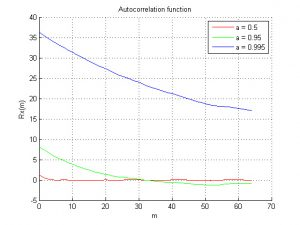MATLAB Handle Graphics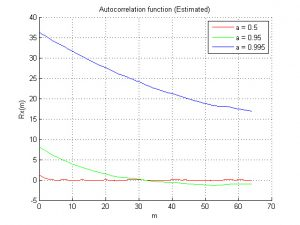MATLAB Handle Graphics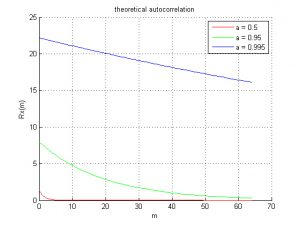MATLAB Handle Graphics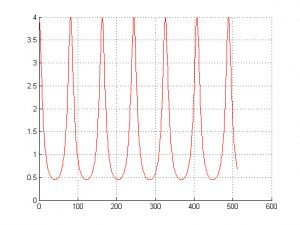MATLAB Handle Graphics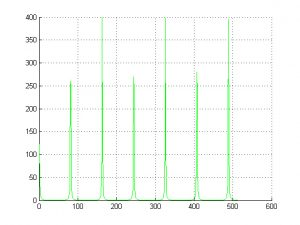MATLAB Handle Graphics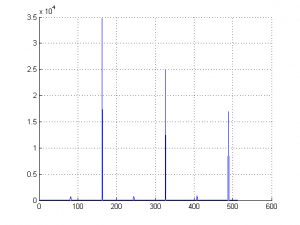MATLAB Handle Graphics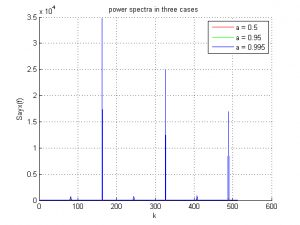MATLAB Handle Graphics

## Generation of random sequences and Time sequences için 1 inceleme

1.5 üzerinden 5 oy aldı